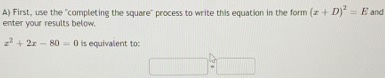### ¿Todavía tienes preguntas de matemáticas?

Pregunte a nuestros tutores expertos
Algebra
PreguntaA) First, use the "completing the square" process to write this equation in the form $$( x + D ) ^ { 2 } = E$$ and enter your results below. $$x ^ { 2 } + 2 x - 80 = 0$$ is equivalent to: ______?